Courses
Courses for Kids
Free study material
Free LIVE classes
More

# How Many Milliliters in a Litre?## Introduction to Capacity and Volume

In ancient times, people used different tools for measuring capacities and volumes. So, there were no standard tools to be used by everyone; they used everyday objects such as jars and pots to measure volume and capacity. So, humans realised a unique system for measurement must be developed to be used worldwide.

Sometimes the amount of liquid inside different bottles is the same, but the capacities of the bottles are different. This amount is called volume. Volume represents the amount of liquid or how much space the liquid takes up in a container. In this article, we will find the relation between various liquid capacities like litres and millilitres and the conversion between these units.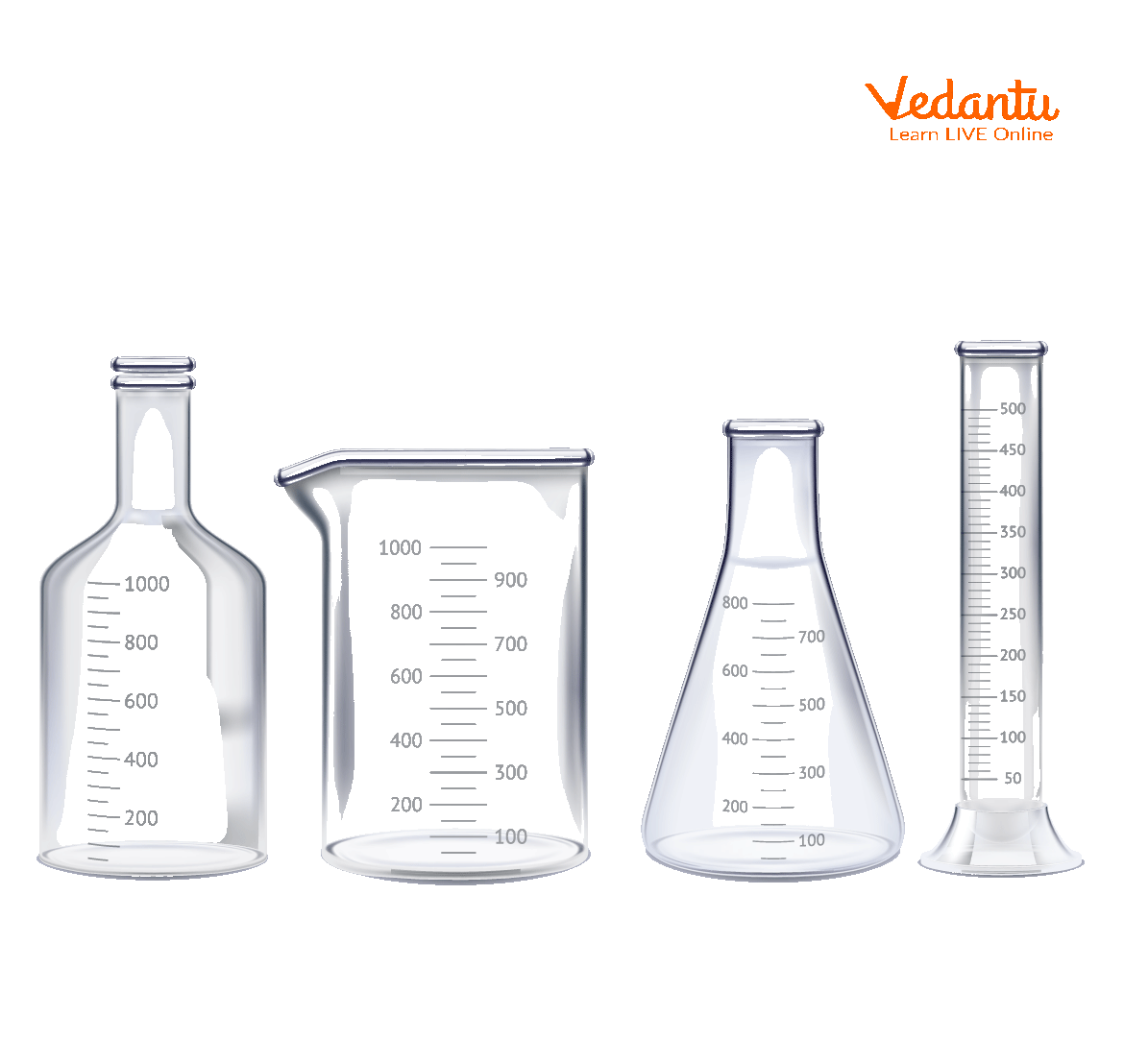Glasswares

## Litres to Milliliters Conversion

Litres and millilitres are metric units of capacity, that is volume used to measure the capacity of a liquid. The capacity of a liquid can be measured in millilitres, centilitres, litres, and kiloliters, according to their capacity to contain volume.

The interesting fact is that all these units represent the same quantity, but their values are different from each other. In this article, we will learn that 1L is equal to how many ml.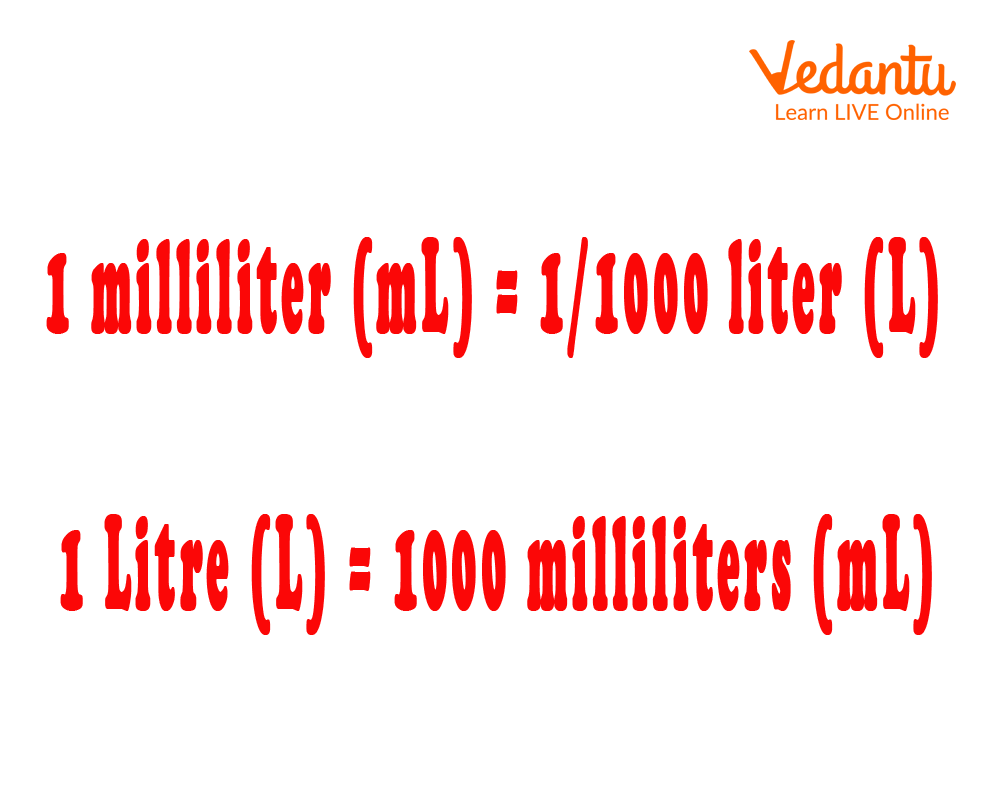Litre to Millilitre Conversion

## What Do You Mean By Litre?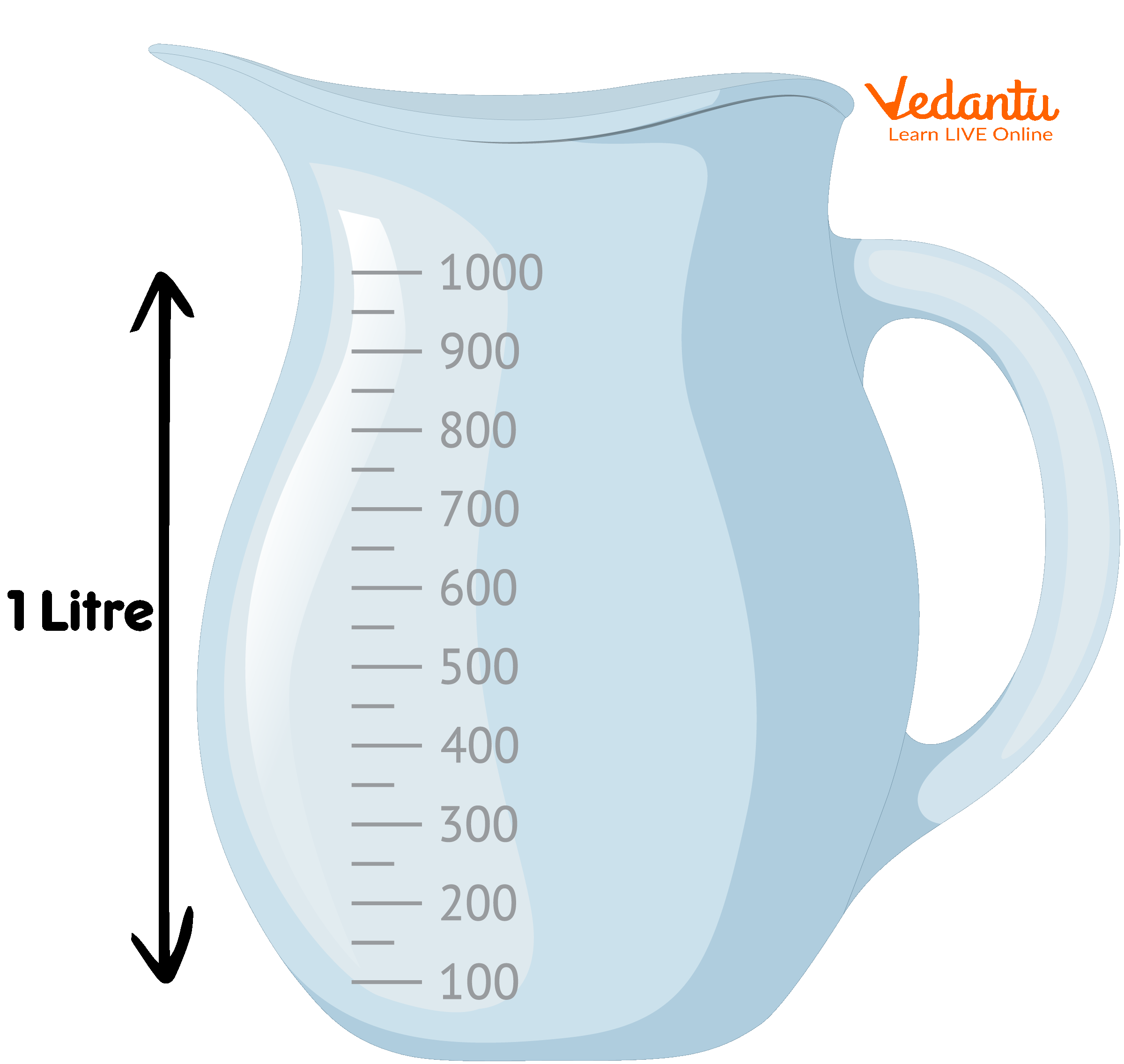Litre Jar

A litre is a basic metric unit used to measure the capacity of liquids and gases, and it is equal to one cubic decimeter (1 litre = 1 dm³). The term litre is denoted by the letter 'l' or L.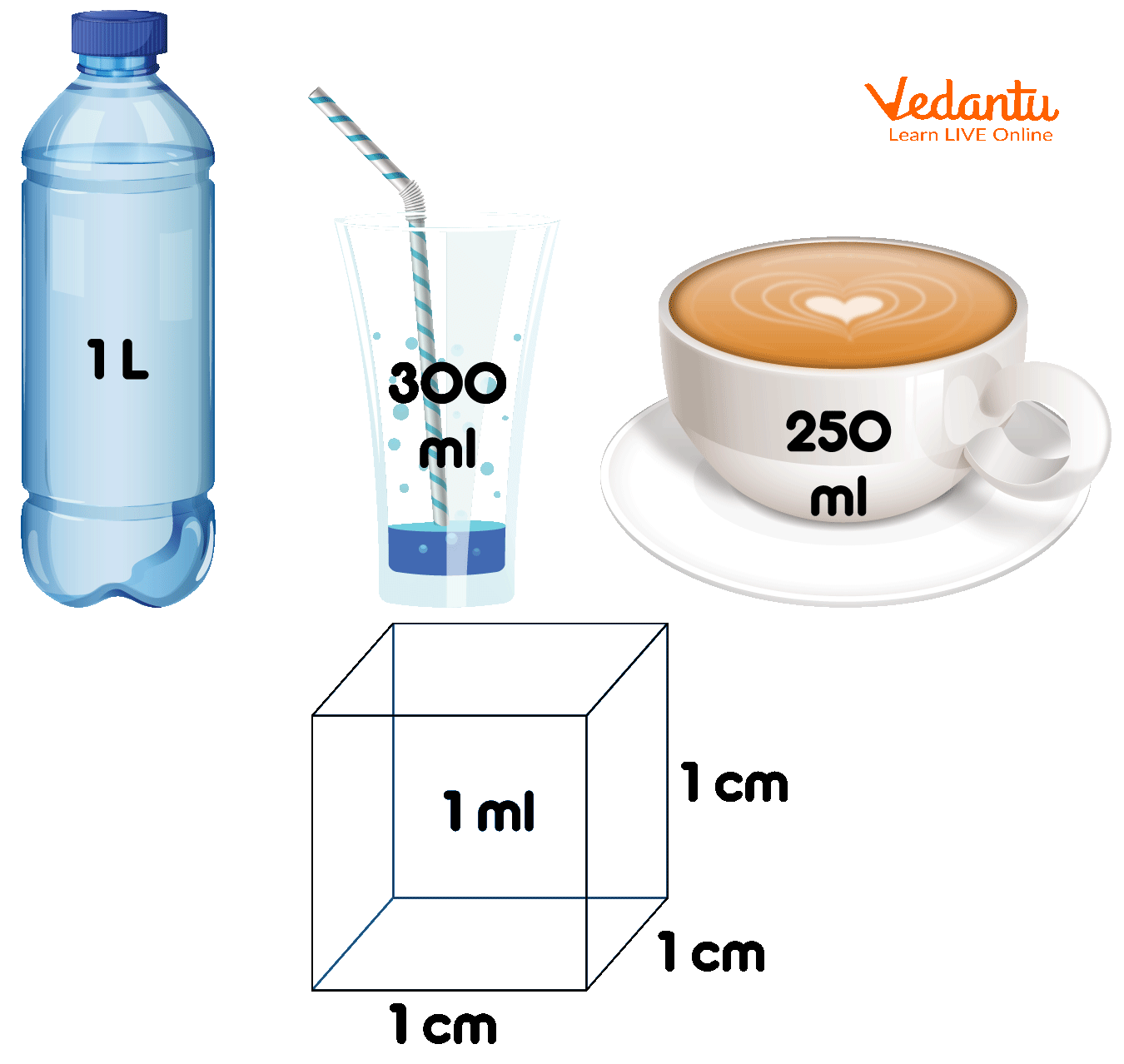Different Measurements Tools of Litre

## What is Milliliter?

• A millilitre is a smaller unit than a litre.

• It represents the volume or the capacity of a liquid.

• It is used to measure a smaller quantity of liquid and is equal to a thousandth of a litre (1 litre = 1000 milliliters).

• A millilitre is denoted by the ml or mL.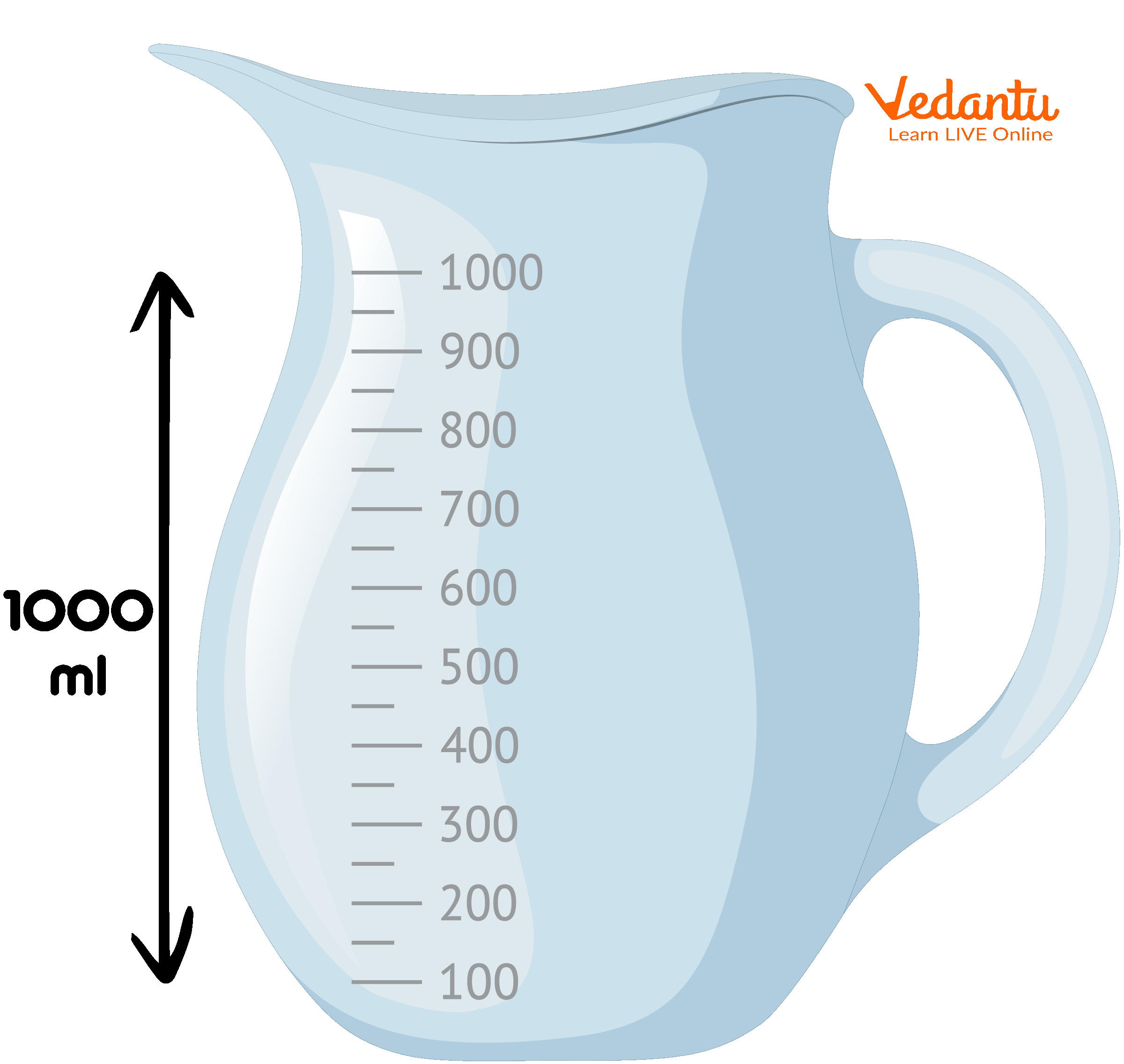Millilitre Jar

## Different Types of Tools Used to Measure Volume of a Liquid

In Maths and Science, the “tools” are used for measuring the volumes of liquids. These tools are made from glass, plastic, and also metal, and if we talk about professional language, these are called “glassware.” In the laboratory, we have seen a variety of glassware used for measuring volumes.

Beakers and flasks are used to make measurements of volumes, volume levels are printed on the side of the beaker.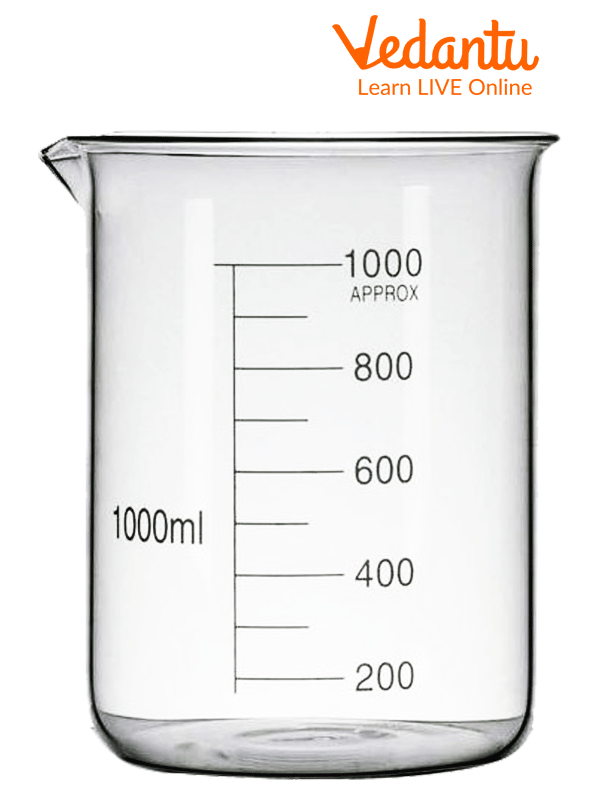Beaker

### Cylinders

• Cylinders are transparent cylinders with divided markings.

• These cylinders are better than beakers and flasks.

• Cylinders are manufactured in sizes ranging from 5 mL to 2000 mL.

• Compared to beakers and flasks, cylinders are available in either glass or plastic, and glass is easier to clean.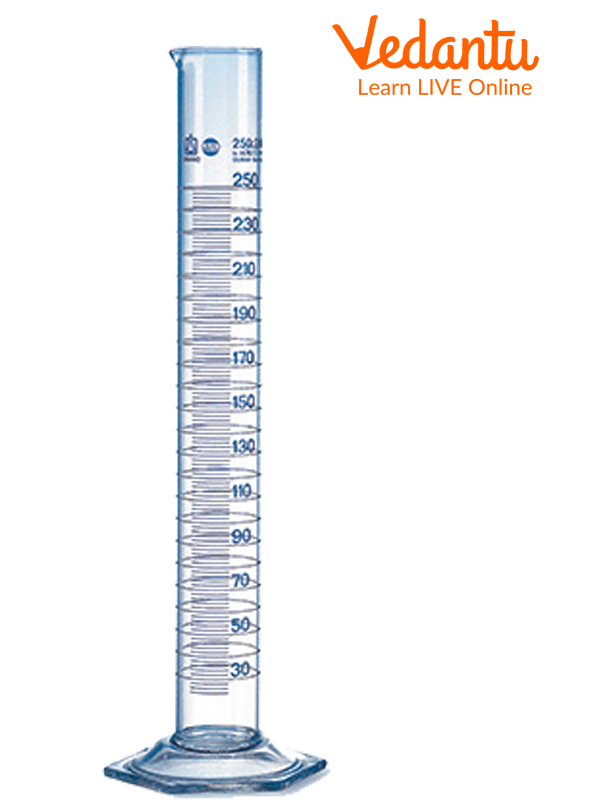Cylinders

### Burette

• Burets are cylindrical pieces of glassware painted on the side.

• Burets are available in sizes from 10 mL to 100 mL; 50 mL is the most common size.

• It is also called a “stopcock” that allows the liquid to flow out through the bottom.

• A large difference exists between a volume of 25 millilitres (shortly mL) and 25.00 mL.

• The measuring device only needs to be able to measure an actual volume like 1 mL.

• Measuring 25.00 mL requires a device capable of measuring within a few one-hundredths of a millilitre.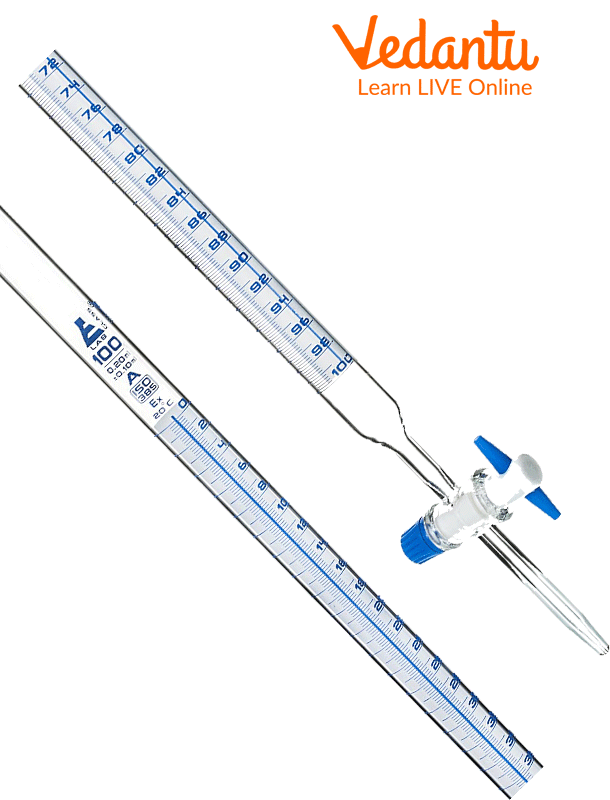Burette

### Pipette

• A pipette is a long tube used to measure out small amounts of solution only.

• It can hold volumes of up to 100 millilitres.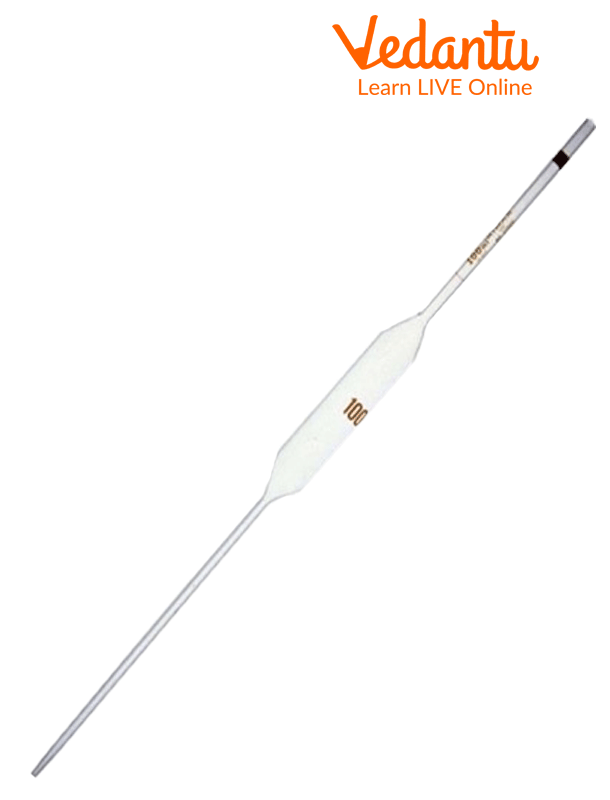Pipette

## How to Convert Liters into Milliliters?

Step 1: The first question that arises is that 1L is equal to how many ml. We all know that 1 litre is equal to 1000 ml. So, the conversion that is used to convert litres to millilitres is 1000.

Step 2: To convert litres to millilitres, we multiply the given quantity by 1000.

Suppose we convert 8 litres to millilitres. So, 8 × 1000 = 8000 ml. Therefore, 8 litres = 8000 millilitres.

Step 3: It should be noted that to convert millilitres to litres, we have to divide the given number by 1000.

Example: Convert 5000 millilitres to litres. So, 5000 ÷ 1000 = 5 liters. Therefore, 5000 millilitres = 5 litres.

Unit conversion is an important part of measurement in maths subjects. Units are converted using the correct conversion of the quantity.

For example, 1 litre is equal to 1000 millilitres, 0.264 gallons, 1.0566 quarts, and 2.1133 pints.

## Solved Examples

Example 1. Convert 200 L to mL.

Ans:

We know that 1 L = 1000 mL

So,

200 L = 200 × 1,000 = 2,00,000 mL

Example 2. Reena bought 4L of tomato juice. So, how many mL of juice does Reena have now?

Ans:

We know that 1 L = 1000 mL

So,

4L = 4× 1000mL

= 4000mL

Reena has 4000 mL juice now.

## Practice Questions

Q1. Convert the given quantity from litres to millilitres -

(a) 5L 400ml

Ans: 5400ml

(b) 7L 600ml

Ans: 7600ml

Q2. Convert the given quantity from millilitres to liter -

(a) 1900ml

Ans: 1.9L

(b) 2700ml

Ans: 2.7L

Last updated date: 01st Oct 2023
Total views: 124.8k
Views today: 3.24k

## FAQs on How Many Milliliters in a Litre?

1. What are some uses of a burette in the laboratory?

Burette is a measuring apparatus used in laboratories to measure the volume of a liquid or a gas. It constitutes a glass tube with a stopcock on top of it. It is commonly used in the titration process for quantitative analysis in many industrial chemical tests where solutions of known concentration are used to find the concentration of unknown solutions. Burettes can be specified according to their volume, resolution, and accuracy of dispensing. Smaller diameter tubes result in lower volume and increased resolution. Digital burettes can achieve better accuracy and are less dependent on the skill of the technician.

2. What are the different types of tools used to measure the volume of a liquid?

In Science, the “tools” for measuring the volumes of liquids are generally made from glass, plastic, or occasionally metal, although professionals referred to all of them as “glassware.” Scientists, and chemists, in particular, have a variety of glassware at their disposal for measuring volumes. The particular piece of glassware chosen in any situation will depend primarily upon two factors: the required volume and the accuracy required for the measurement. Some common tools are listed below:

1. Beakers